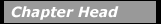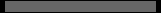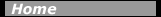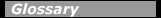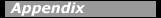## Other SymmetriesIn addition to the symmetries described above, nuclear scientists use a number of other approximate symmetries to describe and predict the behavior of nuclei. Examples of these are charge independence, the expectation that, at the nuclear level, neutron-proton systems should behave the same as proton-proton or neutron-neutron systems; and charge symmetry, the expectation that the interactions between two neutrons should be the same as that between two protons. Charge symmetry can be demonstrated by comparing "mirror nuclei," two low-mass nuclei that have their neutron and proton numbers interchanged and which have very similar nuclear structure, for example 13C (Z=6, N=7) and 13N (Z=7, N=6).

A related symmetry is isospin symmetry, which is related to interchanging the roles of neutrons and protons in certain nuclei. These three symmetries are destroyed when the Coulomb force becomes sufficiently strong, but nevertheless they have proved to be useful approximations in many areas of nuclear science.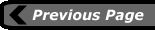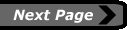last updated: August 9, 2000 webmaster